# Closed-Loop Systems Model Estimation Methods (System Identification Toolkit)

Systems in many real-world applications contain feedback. Feedback is a process in which the output signal of a plant is passed, or fed back, to the input to regulate the next output. Systems without feedback are open-loop systems. Systems with feedback are closed-loop systems.

In an open-loop system, the stimulus signal and the output noise do not correlate with each other. In a closed-loop system, the stimulus signal correlates to the output noise. Though you can apply many open-loop model estimation methods to closed-loop data, not all open-loop model estimation methods handle the correlation between the stimulus signal and output noise well.

## Feedback in Systems

Feedback is common in control systems. With feedback, the system output corresponds to a reference input. Feedback also reduces the effect of input disturbances. One example of a closed-loop system is a system that regulates room temperature, as shown in the following figure. In this example, the reference input is the temperature T set at which you want the room to stay. The thermostat senses the actual temperature, T actual, of the room. Based on the difference between T actual and T set, the thermostat activates the heater or the air conditioner. The thermostat returns T actual as the feedback to compare again with T set. Then the thermostat uses the difference between T actual and T set to regulate the temperature at the next moment.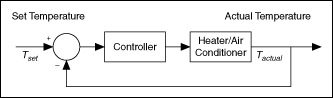You must verify if feedback exists before choosing a model estimation method because not all open-loop model estimation methods work correctly with closed-loop data.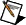Note  You must know whether the data you collect is from an open-loop system or a closed-loop system according to the real-world system configuration. If you do not have such information, you can determine if feedback exists by using the SI Detect Feedback VI or by obtaining the impulse responses of a plant. You can use the Least Squares instances of the SI Estimate Impulse Response VI to estimate the impulse response of a plant.

The following figure shows a comparison of the impulse responses of the plant in a closed-loop system and an open-loop system.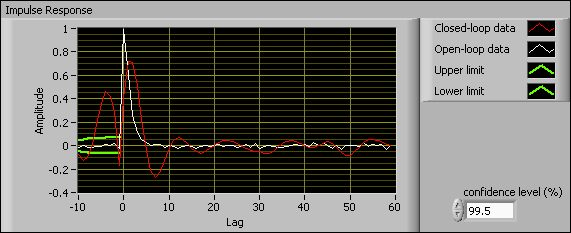The values outside the upper limit and lower limit range at the negative lag, which appears between –10 and 0 on the x-axis, are considered significant values. Significant values in the impulse response at negative lags imply feedback in data. As shown in the following figure, significant values exist in the Closed-loop data plot. Therefore, feedback exists in the closed-loop system. No significant impulse response values exist in the Open-loop data plot. Thus, feedback does not exist in the open-loop system.

## Understanding Closed-Loop Model Estimation Methods

Closed-loop model estimation methods use data from a closed-loop system to build a model for a plant that a controller regulates. The following figure shows a system that consists of a plant and a controller. In this system, G 0 is the plant, F y is the controller, H is the stochastic part of the plant, u is the stimulus signal, y is the response signal, r is the reference signal that is an external signal, and e is the output noise. In control engineering, this system is known as a feedback-path closed-loop system, which is a typical closed-loop system.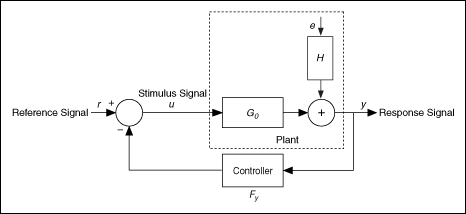In some cases, the controller comes before the plant in a closed-loop system. This system is known as a feedforward-path closed-loop system, as shown in the following figure.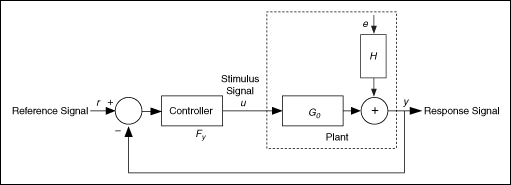Depending on the amount of prior knowledge you have about the feedback, the controller, and the reference signal of a system, you can categorize closed-loop model estimation approaches into the following three groups:

• Direct identification—Uses the stimulus and response signals to identify the plant model as if the plant is in an open-loop system. You can apply the direct identification approach to compute all types of models except state-space models by using the LabVIEW System Identification Toolkit.
• Indirect identification—Identifies a closed-loop system by using the reference signal and the response signal and then determines the plant model based on the known controller of the closed-loop system. You can apply the indirect identification approach to compute transfer function models.
• Joint input-output identification—Considers the stimulus signal and the response signal as outputs of a cascaded system. The reference signal and the noise jointly perturb the system, and the plant model is identified from this joint input-output system. You can apply the joint input-output identification approach to compute transfer function models.

You can choose a suitable model identification approach according to the information you have about the closed-loop system. The following table summarizes the information you must have to use each identification approach.

Stimulus Signal Response Signal Reference Signal Controller Information
Direct X X
Indirect X X X
Joint Input-Output X X X

With the LabVIEW System Identification Toolkit, you can choose to use the direct, indirect, or joint input-output identification approaches for different types of closed-loop systems. The direct identification approach supports single-input single-output (SISO), multiple-input single-output (MISO), and multiple-input multiple-output (MIMO) systems. The indirect and joint input-output identification approaches support SISO systems only.